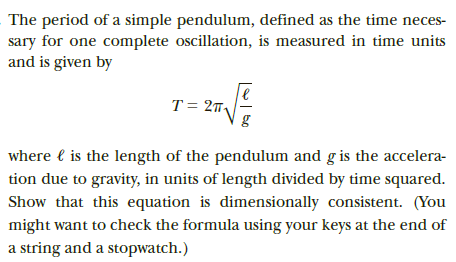# The period of a simple pendulum, defined as the time neces-sary for one complete oscillation, is measured in time unitsand is given byT = 27,where l is the length of the pendulum and g is the accelera-tion due to gravity, in units of length divided by time squared.Show that this equation is dimensionally consistent. (Youmight want to check the formula using your keys at the end ofa string and a stopwatch.)

Question
1 viewshelp_outlineImage TranscriptioncloseThe period of a simple pendulum, defined as the time neces- sary for one complete oscillation, is measured in time units and is given by T = 27, where l is the length of the pendulum and g is the accelera- tion due to gravity, in units of length divided by time squared. Show that this equation is dimensionally consistent. (You might want to check the formula using your keys at the end of a string and a stopwatch.) fullscreen
check_circle

Step 1

Equation for time period of simple pendulum is,

Step 2

Square the equation on both sides.

Step 3

Dimension of T2 is,...

### Want to see the full answer?

See Solution

#### Want to see this answer and more?

Solutions are written by subject experts who are available 24/7. Questions are typically answered within 1 hour.*

See Solution
*Response times may vary by subject and question.
Tagged in

### Units and Dimensions